# Sorting and Filtering Functions

Excel Functions: Excel 365 provides the following sorting and filtering array functions.

SORT(R1, sortcol, order): returns an array with the data in R1 in sorted order based on the elements in column sortcol (the “sort key”) of R1 (default 1). If order = 1 (default), the sort is in ascending order; if order = -1, the sort is in descending order.

UNIQUE(R1): returns an array with the unique entries in the column array R1.

FILTER(R1, criteria, if_empty): returns an array with the subset of the data in R1 that meets the criteria; if none of the data meets the criteria then the if_empty value is returned (if omitted then a #CALC! error value is returned)

E.g. to sort the data in Figure 1 of Sorting and Filtering and place the output in the range starting in cell F4, insert the dynamic array formula =SORT(A4:D12,4) in cell F4 and press Enter. The result is shown in Figure 1.Figure 1 – SORT function

Placing the dynamic array formula =UNIQUE(D4:D12) in cell K4 returns the output shown in column K of Figure 2. Placing the dynamic array formula =SORT(UNIQUE(D4:D12)) in cell M4 returns the output shown in column M of Figure 2.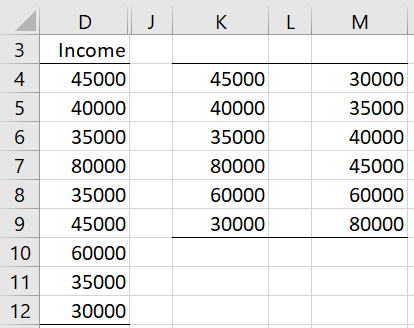Figure 2 – UNIQUE function

We can place the formula

=FILTER(A4:D12, D4:D12>=35000)*(D4:D12<=45000),””)

in cell O4 to extract all the people from Figure 1 who have income between 35,000 and 45,000 inclusive, as shown in Figure 3.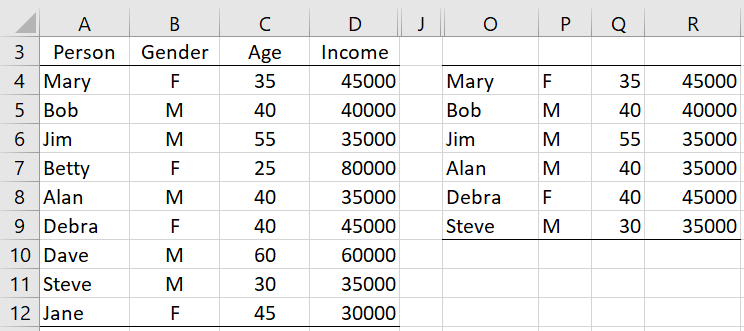Figure 3 – FILTER function (AND criteria)

Note that we use “*” to specify that two criteria must both hold (i.e. as an AND of the two criteria). We can use “+” to specify that one of two criteria must hold (i.e. as an OR of the two criteria). We can use a combination of one or more “+” and “*” to specify more involved criteria.

We can place the formula

=FILTER(A4:D12,(D4:D12>40000)+(B4:B12=“F”),””)

in cell T4 to extract all the entries from Figure 1 of people who have income that exceeds 40,000 or are female, as shown in Figure 4.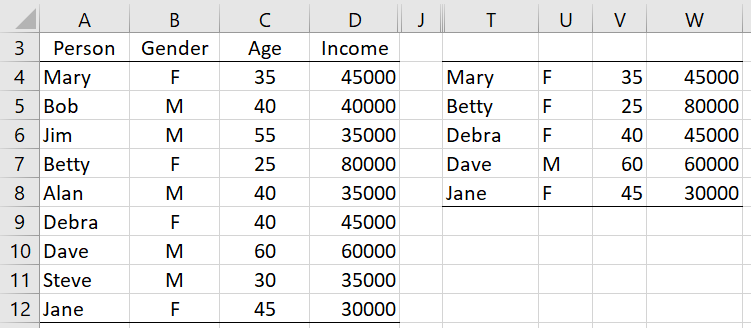Figure 4 – FILTER function (OR criteria)

Note that the criteria expression must refer to a complete row or column or subrange of the range specified in the first argument in FILTER. Thus, while you can use =FILTER(A4:D12,D4:D12>40000) you can’t use =FILTER(A4:D12,D4:D10>40000).

Excel Function: Excel 365 also provides the following enhanced sorting function:

SORTBY(R1, sortcol1, order1, sortcol2, order2, …): returns an array with the data in R1 in sorted order based on the elements in the column array sortcol1 (the “sort key”) of R1 (default 1). If order1 = 1 (default), the sort is in ascending order; if order1 = -1, the sort is in descending order. If specified via sortcol2 you can also use a secondary sort key (and additional sort keys  sortcol3, etc. if you like).

Whereas the sortcol argument in SORT is the index to a column in R1, in SORTBY sortcol1 is a column array or range that may or may not be part of R1, although it must have the same number of rows as R1.

E.g. if you want to sort the data in Figure 1 by income and then by the person’s name (as in Figure 5 of Sorting and Filtering), you can enter the formula =SORTBY(A4:D12,D4:D12,1,A4:A12,1) in cell Y4 and press Enter, as shown in Figure 5.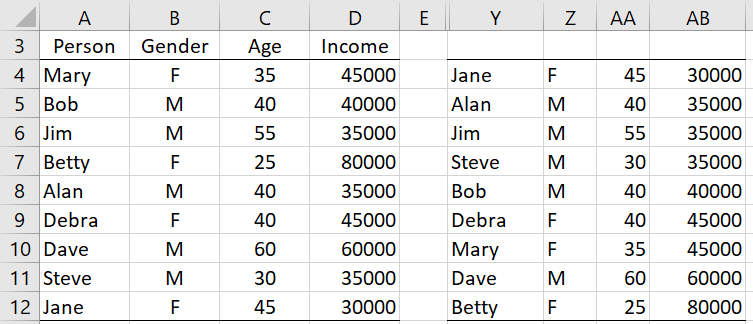Figure 5 – SORTBY function

If you want to sort first by age in ascending order and then by income in descending order but you don’t want the incomes to appear in the output, you can place the formula =SORTBY(A4:C12,C4:C12,1,D4:D12,-1) in cell AD4 and press Enter, as shown in Figure 6.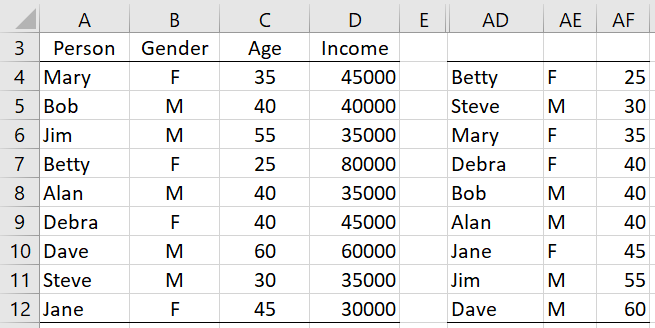Figure 6 – Another SORTBY example

Statistics for Beginners – Introduction to Excel Sorting and Filtering

## Statistics for Beginners – Introduction to Excel Sorting and Filtering Functions

#### Free Machine Learning & Data Science Coding Tutorials in Python & R for Beginners. Subscribe @ Western Australian Center for Applied Machine Learning & Data Science.

Introduction to Applied Machine Learning & Data Science for Beginners, Business Analysts, Students, Researchers and Freelancers with Python & R Codes @ Western Australian Center for Applied Machine Learning & Data Science (WACAMLDS) !!!

Latest end-to-end Learn by Coding Projects (Jupyter Notebooks) in Python and R:

Applied Statistics with R for Beginners and Business Professionals

Data Science and Machine Learning Projects in Python: Tabular Data Analytics

Data Science and Machine Learning Projects in R: Tabular Data Analytics

Python Machine Learning & Data Science Recipes: Learn by Coding

R Machine Learning & Data Science Recipes: Learn by Coding

There are 2000+ End-to-End Python & R Notebooks are available to build Professional Portfolio as a Data Scientist and/or Machine Learning Specialist. All Notebooks are only \$79.95. We would like to request you to have a look at the website for FREE the end-to-end notebooks, and then decide whether you would like to purchase or not.

Please do not waste your valuable time by watching videos, rather use end-to-end (Python and R) recipes from Professional Data Scientists to practice coding, and land the most demandable jobs in the fields of Predictive analytics & AI (Machine Learning and Data Science).

The objective is to guide the developers & analysts to “Learn how to Code” for Applied AI using end-to-end coding solutions, and unlock the world of opportunities!

`Disclaimer: The information and code presented within this recipe/tutorial is only for educational and coaching purposes for beginners and developers. Anyone can practice and apply the recipe/tutorial presented here, but the reader is taking full responsibility for his/her actions. The author (content curator) of this recipe (code / program) has made every effort to ensure the accuracy of the information was correct at time of publication. The author (content curator) does not assume and hereby disclaims any liability to any party for any loss, damage, or disruption caused by errors or omissions, whether such errors or omissions result from accident, negligence, or any other cause. The information presented here could also be found in public knowledge domains.`